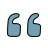### Comparison Of Various Methods Used İn Solving Missing Data Problems İn Terms Of Psychometric Features Of Scales And Measurement Results Under Different Missing Data Conditions

###### Author:

Number of pages:
Year-Number: 2015-Volume 7, Issue 4

### Abstract

In this research, five different methods used in solving missing data problems (listwise deletion, series mean, mean of nearby points, multiple imputation and regression imputation) were compared under the conditions of missing completely at random mechanism (MCAR), normal distribution, unidimentionality, different sample sizes (n=150; n=650) and different missing data rates (%5; %10; %20). The comparisons were made within the context of the psychometric features (eigenvalue; explained variance; Cronbach alpha) of the scale used in the study as a data collection tool and the measurement results (normal distribution, mean and standard deviation) obtained as a result of use of the scale. In line with the objective of the study, data were deleted from the complete data sets (n=150; n=650) at different rates (%5; %10; %20) and the missing data sets were transformed into new complete data sets with five different methods used in solving missing data problems. The values obtained from new complete data sets in terms of psychometric features and measurement results were compared with the ones obtained from the complete data sets; and inferences were made on which methods can be more applicable under which conditions compared to the others. For the comparisons, descriptive statistics were used for eigenvalues, explained variance and score distribution; Fisher’s z test was used for Cronbach alpha; t test for means and Levene’s test for equality of variances for standard deviation. The findings of the research reveal that, under all the conditions within the scope of the research, multiple imputation and regression imputation methods yielded values equal or the nearest to the ones obtained from complete data sets. However, listwise deletion method gave the farest values but the distances are at a negligible level. In comparions made with Fisher’s z test, t test and Levene’s test for equality of variances, no statistically significant differences were found among the values estimated for missing data methods and complete data sets. Therefore, it was concluded that there are no considerable differences among the methods in terms of applicability to solving the missing data problem.

### Abstract

In this research, five different methods used in solving missing data problems (listwise deletion, series mean, mean of nearby points, multiple imputation and regression imputation) were compared under the conditions of missing completely at random mechanism (MCAR), normal distribution, unidimentionality, different sample sizes (n=150; n=650) and different missing data rates (%5; %10; %20). The comparisons were made within the context of the psychometric features (eigenvalue; explained variance; Cronbach alpha) of the scale used in the study as a data collection tool and the measurement results (normal distribution, mean and standard deviation) obtained as a result of use of the scale. In line with the objective of the study, data were deleted from the complete data sets (n=150; n=650) at different rates (%5; %10; %20) and the missing data sets were transformed into new complete data sets with five different methods used in solving missing data problems. The values obtained from new complete data sets in terms of psychometric features and measurement results were compared with the ones obtained from the complete data sets; and inferences were made on which methods can be more applicable under which conditions compared to the others. For the comparisons, descriptive statistics were used for eigenvalues, explained variance and score distribution; Fisher’s z test was used for Cronbach alpha; t test for means and Levene’s test for equality of variances for standard deviation. The findings of the research reveal that, under all the conditions within the scope of the research, multiple imputation and regression imputation methods yielded values equal or the nearest to the ones obtained from complete data sets. However, listwise deletion method gave the farest values but the distances are at a negligible level. In comparions made with Fisher’s z test, t test and Levene’s test for equality of variances, no statistically significant differences were found among the values estimated for missing data methods and complete data sets. Therefore, it was concluded that there are no considerable differences among the methods in terms of applicability to solving the missing data problem.

### Keywords

• Article Statistics
•Quote